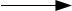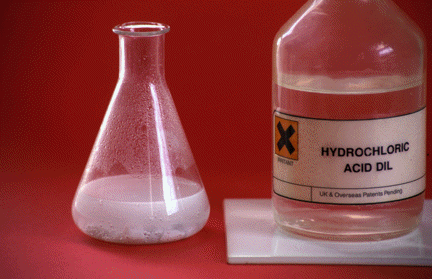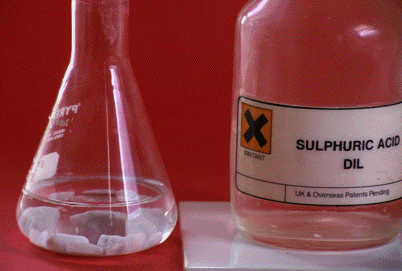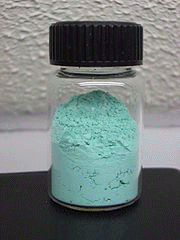Chemguide: Core Chemistry 14 - 16 ``` ``` Reactions between acids and carbonates This page looks at the reactions between acids and carbonates to give a salt, carbon dioxide and water. ``` ``` A summary equation acid + carbonatesalt + CO2 + water ``` ``` Reactions involving calcium carbonate The commonest carbonate-acid reaction you will come across is that between calcium carbonate and dilute hydrochloric acid. Calcium carbonate occurs naturally as chalk, limestone and marble. The photo shows the reaction with marble chips.You get immediate fizzing with a colourless gas given off - that's carbon dioxide. The marble reacts to give a colourless solution of calcium chloride. CaCO3(s) + 2HCl(aq)CaCl2(aq) + CO2(g) + H2O(l) The reaction with dilute sulfuric acid is slightly more complicated because the calcium sulfate formed is only very slightly soluble. The reaction starts, but almost immediately stops again because the marble chips get coated with a layer of calcium sulfate which prevents any more acid getting at the marble chip. The photo shows what the reaction looks like a short time after you have added the acid. Nothing is happening.``` ``` The ionic equation for the reaction All carbonates react in the same sort of way and that is because the same underlying bit of chemistry happens in each case. Carbonate ions from the carbonate react with hydrogen ions from the acid. CO32-(s or aq) + 2H+(aq)CO2(g) + H2O(l) The "(s or aq)" is because a few carbonates (sodium, potassium and ammonium carbonates) are soluble in water, and so you might use a solution of one of these. So if you added dilute hydrochloric acid to sodium carbonate solution, you would again get carbon dioxide produced - but this time everything would be in solution. So what happens to the other ions? They are spectator ions. In the hydrochloric acid / calcium carbonate case, the chloride ions are there in solution all the time. The calcium ions were originally present in the solid and end up in the solution, but they are still calcium ions. So the solution contains calcium ions and chloride ions - calcium chloride solution. ``` ``` The reaction between copper(II) carbonate and dilute sulfuric acidCopper(II) carbonate is an insoluble green powder. This photo comes from Wikipedia. The next video shows its reaction with dilute sulfuric and, and shows how you can test for the carbon dioxide given off. ``` ``` CuCO3(s) + H2SO4(aq)CuSO4(aq) + CO2(g) + H2O(l) The ionic equation, showing the reaction between the carbonate and hydrogen ions, is exactly the same as before - except, of course, that we know copper(II) carbonate is a solid. CO32-(s) + 2H+(aq)CO2(g) + H2O(l) This time the spectator ions you are left with are copper(II) ions and sulfate ions in solution - blue copper(II) sulfate solution. Testing for the carbon dioxide You test for carbon dioxide using lime water - a very dilute solution of calcium hydroxide in water. Lime water is a colourless solution and turns cloudy ("milky") when carbon dioxide is passed through it. The calcium hydroxide reacts with the carbon dioxide to give insoluble calcium carbonate - that's what causes the cloudiness. Ca(OH)2(aq) + CO2(g)CaCO3(s) + H2O(l) ``` ``` Hydrogencarbonates Making calcium hydrogencarbonate If you pass carbon dioxide through lime water for a long time, it first goes milky because of the formation of a precipitate of calcium carbonate, but then the precipitate disappears again giving a colourless solution. The next bit of video shows this happening. The video is a bit clumsy but is chemically accurate. The calcium carbonate precipitate reacts with more carbon dioxide to form calcium hydrogencarbonate, Ca(HCO3)2. Calcium hydrogencarbonate is soluble in water. CaCO3(s) + CO2(g) + H2O(l)Ca(HCO3)2(aq) This is the same reaction which produces cave systems in limestone rocks. Limestone is a form of calcium carbonate. Carbon dioxide dissolved in rain water gradually dissolves the rock over very long periods of time. The reaction of hydrogencarbonates with acids You will find compounds like sodium hydrogencarbonate also called "sodium hydrogen carbonate" (separate words) or the old name "sodium bicarbonate". I prefer the name "sodium hydrogencarbonate" because it shows that the hydrogen and carbonate are both part of the same HCO3- ion. There are very few solid hydrogencarbonates - the only ones you are likely to meet are sodium and potassium hydrogencarbonates. Calcium hydrogencarbonate only exists in solution. If you try to turn it into a solid, it splits up again into calcium carbonate and carbon dioxide and water. Hydrogencarbonates react with acids in the same way as carbonates. So, for example, if you add dilute hydrochloric acid to solid sodium hydrogencarbonate, it will react giving off colourless carbon dioxide gas and producing colourless sodium chloride solution. NaHCO3(s) + HCl(aq)NaCl(aq) + CO2(g) + H2O(l) The ionic equation for the reaction is HCO3-(s) + H+(aq)CO2(g) + H2O(l) Note:  To be honest, at this level you might come across calcium hydrogencarbonate being formed in the lime water reaction, and you might come across sodium hydrogencarbonate being used to test for an acid. If you add solid sodium hydrogencarbonate to a liquid and get carbon dioxide given off, then the liquid must be acidic. You might also come across calcium or magnesium hydrogencarbonates if you do a topic about hard water. And that is about all you will need to know! ``` ``` Where would you like to go now? To the acid, bases and salts menu . . . To the Chemistry 14-16 menu . . . To Chemguide Main Menu . . . ``` ``` © Jim Clark 2020